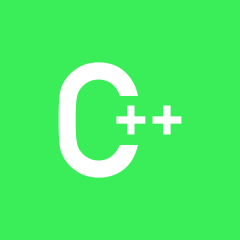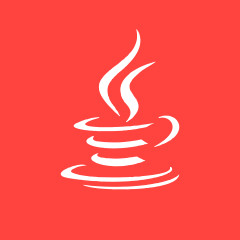### C++C++ JAVA AndroidC++ Html/CSS JavaScriptC++ Html/CSS PHPC C# C++Android C++ iOSC C++ JAVAC++ Html/CSS JAVAJAVA C++C C++ PythonC++
q－>next=NULL；//这句有什么用C C++ 数据结构C++ JAVA PHPC++C C# C++
Web前端，应该从哪里学起C C++
c语言变长数组和动态数组有什么区别，能否完全取代？C C++ C#C++C C++ LinuxAndroid C++ MySQL
c语言 求和公式的程序 成功 但是警告9个 无法执行 QAQ？C C++C C++ JAVAC C++C C++ JAVAC 数据结构 C++WebApp C++ C#
C++闪退退退退退退啊啊！怎么办？C++
mysql为什么没有配置文件C++ JAVA MySQL
c语言大家都用啥软件开发C C# C++C C++ JAVAC C++ JAVA